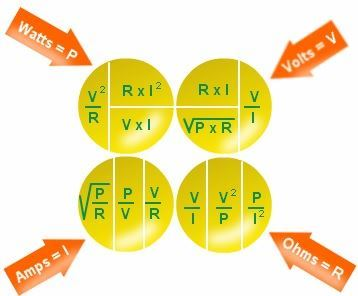# Electricity Formulas

## Electricity Formulas

Electricity is the flow of charge in a conductor from anode to cathode. Electricity has varied applications It acts as a tool to provide power to electrical devices. We can say the flow of charge builds up the current which we call as Electricity. To understand how the Electricity is generated, we need to understand the various basic parameters related to it like voltage, Current, resistance, conductivity and relation among them.Some of the commonly used Electricity formula are listed below.

(TABLE NEEDS TO BE CREATED)

 Quantity Formulas Unit Current I I = Q / t Q = Charge t = time taken Amperes (A) Voltage V V = E / Q or V = W / Q E = Energy, W = Work done Volts (V) Resistance R R = ρl / A ρ = Resistivity, l = length, A = Area Ohm (Ω) Resistance R R = V / I ohm (Ω) Power P P = VI Watts (W) Conductivity σ sigma = 1 / ρ Siemens per meter (S/m)

Electricity Formulas are applied in calculating the unknown electrical parameters from the known in electric circuits.

Example 1

Determine the current flowing through the electric heater have p.d of 220 V and resistance is 70 Ω.

Solution:

Given:

Resistance R = 70 Ω

Voltage V = 220 V

The current formula is given by

I = V / R

= 220 / 70

= 3.1428 A

Example 2

An electrical lamp lights for 4 hours and draws a current of 0.5 A. Calculate the amount of charge flowing through the lamp.

Solution:

Current I = 0.5 A

Time taken t = 4 hours

= 4 × 3600 = 14400 s,

Charge Q = I × t

= 0.5 ×14400

= 7200 C

#### 1 Comment

1. Lochen kumar luitel

Very nicely you are helpful for me coz i understand everything that i know